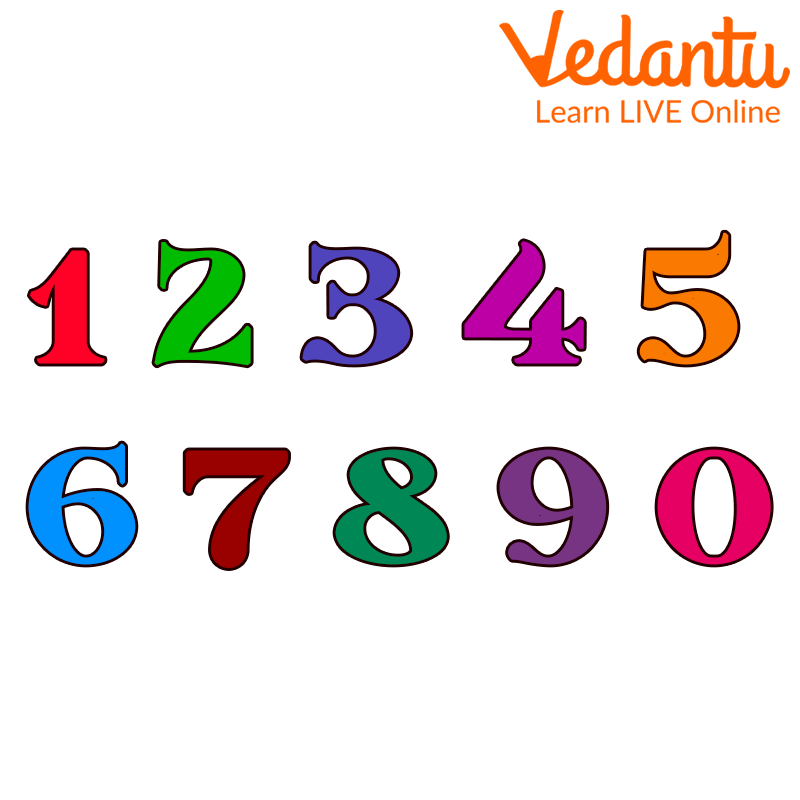Courses
Courses for Kids
Free study material
Free LIVE classes
More

# How to Find the Smallest Number?LIVE
Join Vedantu’s FREE Mastercalss

## How Can We Arrange Numbers in Maths?

The smallest number in a given group of numbers will be the smallest number. Like 1 is the smallest natural number, 0 is the smallest whole number, and so on. The numbers can be arranged in order, ascending or descending. By arranging numbers in ascending order, we will get the smallest number and by arranging numbers in descending order, we will get the greatest number.

## What is a Digit?

A positional numeral system uses a single symbol, the numerical digit, either individually or in combination to represent numbers. The term "digit" refers to the ten symbols of the common base-10 numeral system, or decimal digits, to which the ten digits of the hands correspond. The number from 0 to 9 are called digits. For example, the numeral 321 coonsists of 3, 2, and 1 digit.Digits

## What is the Ascending and Descending Order?

Ascending Order: When numbers or things are arranged from lowest to highest or from small to big, then the order will be ascending order.Arrangement of People in Ascending Order of their Height

Descending Order: When numbers or things are arranged from highest to lowest or from big to small, then the order will be descending order.Arrangement of People in Descending Order of their Height

### How to Form the Smallest Number from given Digits?

• Arrange the given digits in ascending order to get the smallest number.

For example, the given digits are 3, 2, and 4.

Step 1: Arranging in ascending order, we get the smallest number that is from small to big.

Step 2: Thus, its ascending order will be 2, 3, 4.

• If zero is one of the digits when composing a smaller number, it is written after the highest place. For example, given digits are 3, 0, and 5.

The smallest is 305. Because if we make the digit 035, then it would be a 2-digit number which is not required as we have to make the smallest 3-digit number only.

• 0 (Zero) is the smallest whole number.

• 1 (one ) is the smallest natural number.

• Zero is the smallest non-negative rational number and non-negative real number.

• The smallest 5-digit number is 10000.

• The smallest 7-digit number is 1000000.

## Solved Examples

1. Form the 3 smallest digit numbers from the given digits.

1. 2,4 and 5

2. 6,8 and 4

3. 3,7 and 9

Solution:

a. 2,4 and 5

To get the smallest number, arrange them in ascending order; you will get 2,4,5, so the smallest number is 245.

b. 6,8 and 4

To get the smallest number, arrange them in ascending order; you will get 4,6,8, so the smallest number is 468.

c. 3,7 and 9

To get the smallest number, arrange them in ascending order; you will get 3,7,9, so the smallest number is 379.

2. Arrange the following digits in ascending order.

1. 9,4 and 8

2. 3,0 and 7

Solution:

a. 9,4 and 8

Ascending Order: 4,8,9

b. 3,0 and 7

Ascending Order: 0,3,7

3. Arrange the following digits in ascending order.

1. 9,5 and 4

2. 1,0 and 3

Solution:

a. 9,5 and 4

Ascending Order: 4,5,9

b. 1,0 and 3

Ascending Order: 0,1,3

## Conclusion

The smallest number from the digits given can be formed by arranging the digits in ascending order. We put the digits in ascending order to get the smallest number. In this article, we have learned that to obtain the smallest number from the given digits, we need to arrange the numbers in ascending order such that the smallest digit comes at the left-most digit and the largest digit is in the rightmost digit. If there is zero, then it must be at the second digit to the left, because if we place zero at the left-most digit, then it is no longer a three-digit number.

Last updated date: 02nd Oct 2023
Total views: 94.5k
Views today: 0.94k

## FAQs on How to Find the Smallest Number?

1. Where is the concept of smallest numbers used?

• In insurance policies.

• In Medical instruments

• For checking heartbeat rate.

2. Give different names of numbers.

There exist different types of numbers, such as rational numbers, real numbers, imaginary numbers, whole numbers, natural numbers and many more.

3. Write the greatest five-digit and seven-digit numbers.

The greatest five-digit and seven-digit numbers are 99999 and 9999999.